Published 15.01.2003, updated 30.06.2003

ENERGY BALANCE OF FUSION PROCESSES OF MOLECULES OF OXYGEN, HYDROGEN AND WATER

Ph.M. Kanarev

The Kuban State Agrarian University, Department of Theoretical Mechanics

13, Kalinin Street, 350044 Krasnodar, Russia

E-mail: kanphil@mail.kuban.ru

Abstract. A cause of additional energy appearance during covalent bond formation in the fusion processes of the molecules of oxygen and water has been disclosed, and the source of this energy has been established.

Key words: nucleus, atom, molecule, electron, photon, bond

INTRODUCTION

In engineering practice connected with ventilation system servicing, a phenomenon of excessive thermal energy in circulating air has been found. Similar phenomenon has been registered in water circulation systems with the devices for its active cavitations. The results of our investigations explain not only a cause of these phenomena, but they give an opportunity to perform quantitative calculations of energy processes, which generate additional thermal energy , , , , .

THEORETICAL PART

An oxygen atom is the eight element of the periodic table. It is situated in its sixth group. The structure of its nucleus is given in Fig. 1 , , .Fig. 1. Diagram of oxygen atom nucleus: light – the protons, dark and grey – the neutrons

In Fig. 2, a diagram of the oxygen atom originating from the structure of its nucleus is given (Fig. 1). It has eight electrons. The electrons situated on the axis of symmetry are the most active ones (1, 2). Other six electrons situated in the plane, which are perpendicular to an axis line (a line of symmetry), take away electrons 1 and 2 from the nucleus at a large distance by their total electric field forming the conditions for their large activity during the interaction with the electrons of the neighbouring atoms , , .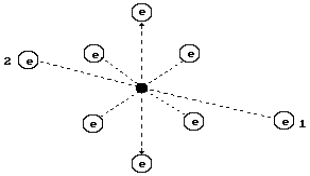Fig. 2. Diagram of the oxygen atom

The least ionization energy of the electron of the oxygen atom is equal to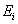=13.618 eV. Binding energy of this electron with the atomic nucleus corresponding to the first energy level is equal to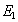=13.752 eV. Let us call this electron the first one. The calculation of energy indices of this electron, including its binding energies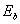with the atomic nucleus according to the formulas (1) and (2), gives the following results (Table 1) , , .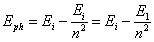(1)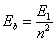(2)

Table 1. Spectrum of the first electron of the oxygen atom

 Values n 2 3 4 5 6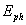(exp.) eV 10,18 12,09 12,76 13,07 13,24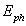(theor.) eV 10,16 12,09 12,76 13,07 13,24(theor.) eV 3,44 1,53 0,86 0,55 0,38

The oxygen molecule structure is given in Fig. 3, a. It is formed by means of a connection of unlike magnetic poles of axis electrons of two oxygen atoms , , . It is known that the fusion process of the oxygen molecules is accompanied with a release of 495 kJ/mole of energy, or in calculation for one molecule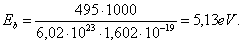(3)

What principle does the nature go by distributing energy 5.13 eV between the oxygen molecule electrons  (Fig. 3, a)? Energy of 5.13 eV is a thermal binding energy between the electrons 1 and 2’ of two oxygen atoms (Fig. 3, a). When the oxygen molecule is formed, it is emitted in the form of the photons by the electrons, which enter the bond. It appears from this that it is equal to an amount of energies of two photons emitted by these electrons. Consequently, each contacting electron emits  a photon with energies of 5.13/2=2.565 eV=(Fig. 3). According to Table 1, in this case the valence electrons are situated between the second energy level and the third one .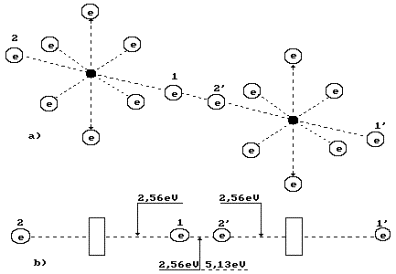Fig. 3. Diagram of binding energy distribution between the electrons in the oxygen molecule

Two oxygen atoms are connected into a molecule in an excitation state. The excitation state is the state of an atom when its valence electrons are situated at such distances from the nuclei when the binding energybetween them is reduced to the thousandth fractions of an electron-volt. In such state, the atom can loose an electron and become an ion. Otherwise, without loosing electrons it is connected with an electron of the neighbouring atom by the valence electron, and a process of oxygen molecule formation begins. It is an exothermic process when the axis valence electrons 1 and 2’ emit the photons, descend on lower energy levels and release 2.565x2=5.13 eV.

Let us pay attention to the fact that energy 5.13 eV is released by two electrons, which form a bond with energy of=2.56 eV. In modern chemistry, this bond is called a covalent bond. In order to break this bond, it is necessary to use 2.56 eV of mechanical energy. For thermal cleavage of this bond, double quantity of energy is required, i.e. 5.13 eV. It is explained by the fact that the photon energy of 5.13 eV is absorbed by two electrons simultaneously. Only in this case, both electrons will be transferred to the highest energy levels with minimal binding energywhen they are disconnected, and each oxygen atom becomes a free one.

Thus, energy expenses for an oxygen molecule destruction depend on an impact method on the bond. During thermal impact on the bond it is destroyed when energy is 5.13 eV. During mechanical impact of the bond, it is necessary to spend 2.56 eV of energy in order to destroy this bond. It appears from this that energetic of fusion process of the oxygen molecule depends on its destruction method.

After the thermal destruction of the oxygen molecule its formation process begins from emission of the photons with energies of 2.56 eV by both valence electrons, and the previous electrodynamics binding energy (=2.56 eV) is restored between the electrons of both atoms.

Thus, during the thermal destruction of the oxygen molecule the same amount of thermal energy is spent, which is released during its further formation. No additional energy appears during thermal dissociation of the oxygen molecule and its further fusion.

If the oxygen molecule is destroyed by a mechanical method, it is necessary to spend 2.56 eV of mechanical energy for this purpose. Valence electrons of the oxygen atoms are in a free state by a lack of energy corresponding to such state as there is no absorption process of 2.56 eV of energy by each of them. The electrons cannot remain in such state, they should replenish immediately the energy, which they have failed to receive during a mechanical break of the bond between them. Where should they take it? There is only one source: environment, i.e. physical vacuum filled with ether. They convert ether into energy of 2.56 eV immediately. The next stage is a connection of two oxygen atoms, which valence electrons have replenished the reserves of their energy at the expense of ether. This process is accompanied by emission of the photons with energies of 2.56 eV by two electrons. Thus, energy of absorbed ether is converted into thermal energy of the photons. If we spend 2.56 eV of mechanical energy for the oxygen molecule destruction, we’ll get double quantity of energy (2.56x2=5.13) eV during further fusion of this molecule. Additional energy is equal to 2.56 eV.

Many experimental data show that in ventilation systems thermal energy of circulating air exceeds electric energy spent for a fan drive. Now we know that this energy is generated at mechanical failure of covalent bonds in the molecules of the gases, which the air consists of.

Using the above-mentioned method, we’ll analyse water molecule energetic, which sometimes generates additional thermal energy. A water molecule consists of one oxygen atom and two hydrogen atoms. Binding energiesof the hydrogen atoms with its nucleus are given in Table 2 , , .

Table 2. Spectrum of hydrogen atom

 Values n 2 3 4 5 6(exp.) eV 10,20 12,09 12,75 13,05 13,22(theor) eV 10,198 12,087 12,748 13,054 13,220(theor.) eV 3,40 1,51 0,85 0,54 0,38

It is known that a connection of hydrogen with oxygen is accompanied by an explosion, but its cause remains unknown. Let us try to find it.

Hydrogen molecule fusion energy is equal to 436 kJ/mole, or 4.53 eV per molecule. As the molecule consists of two atoms, the above-mentioned energy is distributed between them. Thus, energy of one bondbetween the hydrogen atoms is equal to 2.26 eV (Fig. 4). At thermal failure of this bond, double quantity is required 2.26x2=4.53 eV .

In order to form two water molecules, it is necessary to break two hydrogen molecules and one oxygen molecule into atoms. If the destruction processes of the above-mentioned molecules are carried out with a thermal method, 4.53+4.53=9.06 eV are required for the destruction of two hydrogen molecules, and 5.13 eV are required for the destruction of one oxygen molecule. Totally, it is 14.19 eV. The difference between the energy spent for mechanical and thermal destruction of a covalent bond of the molecules of hydrogen and oxygen is almost double.

It is known that during fusion of one mole of water 285.8 kJ or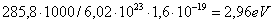per molecule are released. As a water molecule consists of one oxygen atom and two hydrogen atoms, 2.96/2=1.48 eV falls per bond (Fig. 5). It appears from this that the electrons of the atoms of hydrogen and oxygen in the water molecule are at the usual temperature 1.48/2=0.74 eV =between the forth energy level and the fifth one (Table 2) .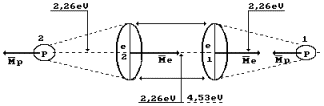Fig. 4. Hydrogen molecule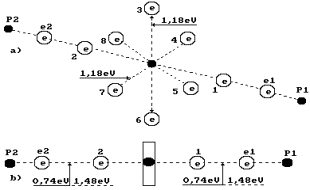Fig. 5. Diagram of water molecule:

1,2,3,4,5,6,7,8 are the numbers of the electrons of the oxygen atom; P1, P2 are the nuclei of the hydrogen atoms (the protons); e1 and e2 are the numbers of the electrons of the hydrogen atoms

Thus, when two hydrogen molecules 2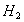and one oxygen molecule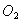are destroyed by the thermal method, 14.19 eV are spent. As a result of fusion of two water molecules (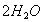), 2.96x2=5.98 eV are released. It conflicts with the fact that water molecule fusion process is an exothermic one with a release of 2.96 eV by one molecule. The given calculation shows that (14.19-5.98)/2=4.10 eV are absorbed during fusion of one water molecule. What is the cause of this contradiction?

The oxygen atom in the water molecule should reduce its volume when the transition from gaseous state into liquid state takes place. It will happen when the rings electrons of the oxygen atom descend on lower energy levels (nearer to the nucleus). They will emit the photons, and we know their total energy. It is equal to energy spent to destruction of two hydrogen molecules and one oxygen molecule, i.e. 14.19 eV. As two water molecules have 12 ring electrons, each of them will emit 14.19/12=1.18 eV=(Fig. 5). It is more than axis electron binding energy (=0.74 eV) with the nucleus, and it shows that the ring electrons are situated nearer to the nucleus than the axis ones.

In this case, quantity of the energy produced due to fusion of two water molecules (14.19+5.98) eV exceeds the energy spent for the destruction of two hydrogen molecules (9.06 eV) and one oxygen molecule (5.13 eV). The formed energy difference of 5.98 eV is divided between two water molecules. It means that 5.98/2=2.99 eV or 285.8 kJ/mole fall per molecule. It corresponds to the existing experimental data completely .

The above-mentioned facts clarify a cause of the explosion, which takes place when hydrogen is combined with oxygen. Simultaneous transition of six ring electrons of each oxygen atom in the nascent water molecules to lower energy levels is accompanied by simultaneous emission of the photons, which generate explosion phenomenon.

Let us pay attention to the fact that two binding energiesbetween valence electrons e2 and 2 and between 1 and e1 are shown in Fig. 5, b. Energy of one electrodynamics bond is equal to= 0.74 eV. If this bond is destroyed by the thermal method, 0.74x2=1.48 eV is required. This energy will be released during further fusion of the water molecule from hydrogen atom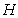and hydroxyl ion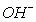. In this case, no additional energy is generated.

It appears from this that the given bond is destroyed by the mechanical method spending 0.74 eV per bond, each electron will have energy deficit equal to 0.74 eV after bond failure. This energy will be absorbed from the environment immediately and will be emitted during the repeated fusion of the water molecule from the hydrogen atom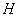and the hydroxyl ion. At mechanical failure of one bond of water molecule, the covalent chemical bond forms= 0.74 eV of additional thermal energy, which is registered in the water cavitation systems constantly (as we have already noted) , , .

It is known that the water molecules unite and form clusters. If the bonds between the molecules in the clusters are covalent ones, mechanical destruction of these bonds should be accompanied by a release of additional thermal energy as well , , .

Experimental Part

Thus, chemical bonds between the atoms in the molecules and the molecules in the clusters can be destroyed mechanically, by electrodynamic and thermal impact on them. We have already shown that the mechanical way of destruction of such bonds requires half energy as compared with thermal energy. It appears from this that energy expenses for electrodynamic destruction of these bonds should be less than thermal expenses as well. Electrodynamic impact on the bond gives the opportunity to form the resonance modes where energy expense for the destruction of these bonds is reduced to greater degree. In order to check this hypothesis, a special experiment was carried out connected with electrodynamic destruction of chemical bonds of water molecules with a changing frequency of impact. The check experiment in order to test this hypothesis was prepared and carried out by (besides the author of this article) A.I. Tlishev, G.P. Perekotiy, D.A. Bebko, D.V. Korneev. A diagram of the experimental installation is given in Fig. 6. The results of this experiment are given in Table 3.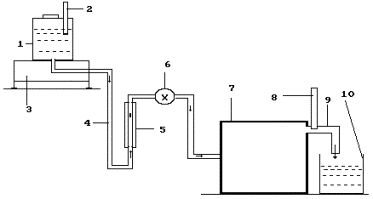Fig. 6. Diagram of the experimental installation: 1 - reservoir for solution; 2- thermometer; 3- electronic scales; 4 - solution feed duct; 5- rotameter; 6- solution feed regulator; 7-a special thin plasma reactor is in the process of patenting; 8 - thermometer; 9- heated solution discharge; 10- inlet reservoir

PROTOCOL  OF CONTROL TEST

 Indices 1 2 3 Mean 1 – mass of the solution, which has passed through the reactor m,  kg. 0.434 0.44 0.434 0.436 2 – temperature of solution at the input of the reactor  t1, degrees 24 24 24 24 3 – temperature of the solution at the output of the reactor t2, degrees 80 80 80 80 4 – temperature difference of the solution Dt= t2 - t1, degrees 56 56 56 56 5 – durability of the experiment  Dt, s 300 300 300 300 6 – reading of voltmeter V, V 20 20 20 20.0 7 – reading of ammeter I, A 1.4 1.4 1.4 1.40 8 – electric power consumption according to indices of voltmeter and ammeters, E2=I×V×Dt, kJ 8.4 8.4 8.4 8.40 9 – power spent for heating of the solution, E3=4.19×m×Dt, kJ 101.83 103.24 101.83 102.30 10 – reactor efficiency index K= E3/ E2 12.12 12.3 12.12 12.18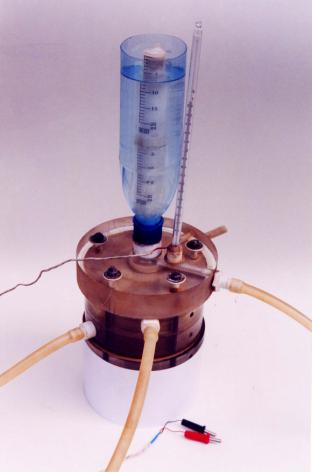Fig. 7. Photo of reactor heart

In the Russian market, three firms (Yusmar, Termovikhr and Noteka) sell cavitation water heating equipment with energy efficiency index of 150%. Soon, an air heating device with the same efficiency will be produced. The processes of mechanical destruction of covalent bonds of the air gas molecules, molecules and clusters of water and their further fusion serve as a source of additional energy generated by these devices , , .

CONCLUSION

Analysis of energy balance of the molecules with covalent bonds shows the possibility of additional thermal energy formation during mechanical and electrodynamics destruction of these bonds.

References

1. Ph.M. Kanarev. The Foundation of  Physchemistry  of  Micro World. Krasnodar. 2002. 320 pages. (In Russian).

2.  Kanarev Ph.M. The Foundation of  Physchemistry of  Micro World. The second edition. (In Russian).   http://www.ikar.udm.ru/sb28-2.htm

3. Kanarev Ph.M. The Foundation of  Physchemistry of  Micro World. The second edition. (In English). http://book.physchemistry.innoplaza.net

4. Kanarev Ph. M. Energy Balance of Fusion Processes of  Molecules of  Oxygen, Hydrogen and Water.  (In English) http://Kanarev.innoplaza.net

5.  Kanarev Ph. M. Energy Balance of Fusion Processes of  Molecules of  Oxygen, Hydrogen and Water. (In Russian) http://www.n-t.org/tp/ts/eb.htm

http://Kanarev.energy.innoplaza.net

<< Kanarev´s Page